# Curvature is antisymmetric in last two variables

(diff) ← Older revision | Latest revision (diff) | Newer revision → (diff)

## Statement

Suppose$M$ is a differential manifold and$g$ is a Riemannian metric or pseudo-Riemannian metric and$\nabla$ is the Levi-Civita connection for$g$. Consider the Riemann curvature tensor$R$ of$\nabla$. In other words,$R$ is the Riemann curvature tensor of the Levi-Civita connection for$g$. We can treat$R$ as a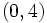$(0,4)$-tensor: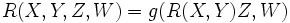$\! R(X,Y,Z,W) = g(R(X,Y)Z,W)$.

Then: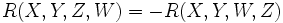$\! R(X,Y,Z,W) = -R(X,Y,W,Z)$.

## Proof

We consider the expression$R(X,Y,Z,W) + R(X,Y,W,Z)$: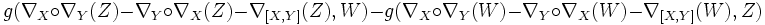$g(\nabla_X \circ \nabla_Y(Z) - \nabla_Y \circ \nabla_X(Z) - \nabla_{[X,Y]}(Z),W) - g(\nabla_X \circ \nabla_Y(W) - \nabla_Y \circ \nabla_X(W) - \nabla_{[X,Y]}(W),Z)$

By the bilinearity of$g$, this simplifies to:$g(\nabla_X \circ \nabla_Y(Z),W) - g(\nabla_Y\circ \nabla_X(Z),W) - g(\nabla_{[X,Y]}(Z),W) + g(\nabla_X \circ \nabla_Y(W),Z) - g(\nabla_Y\circ \nabla_X(W),Z) - g(\nabla_{[X,Y]}(W),Z)$

To prove that this is zero, it thus suffices to show that: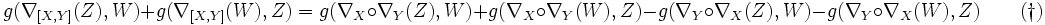$g(\nabla_{[X,Y]}(Z),W) + g(\nabla_{[X,Y]}(W),Z) = g(\nabla_X \circ \nabla_Y(Z),W) + g(\nabla_X \circ \nabla_Y(W),Z) - g(\nabla_Y \circ \nabla_X(Z),W) - g(\nabla_Y \circ \nabla_X(W), Z) \qquad (\dagger)$.

We now show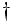$\dagger$. Since$g$ is a metric connection, the left side simplifies to:$g(\nabla_{[X,Y]}(Z),W) + g(\nabla_{[X,Y]}(W),Z) = [X,Y]g(Z,W) = XYg(Z,W) - YXg(Z,W) \qquad (\dagger\dagger)$.

Simplifying each of the two terms on the right side of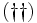$(\dagger\dagger)$, we get: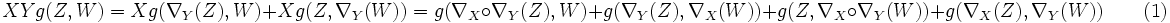$XYg(Z,W) = Xg(\nabla_Y(Z),W) + Xg(Z,\nabla_Y(W)) = g(\nabla_X \circ \nabla_Y(Z),W) + g(\nabla_Y(Z),\nabla_X(W)) + g(Z,\nabla_X \circ \nabla_Y(W)) + g(\nabla_X(Z),\nabla_Y(W)) \qquad (1)$.

And: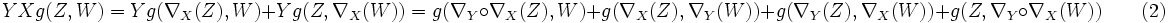$YXg(Z,W) = Yg(\nabla_X(Z),W) + Yg(Z,\nabla_X(W)) = g(\nabla_Y \circ \nabla_X(Z),W) + g(\nabla_X(Z),\nabla_Y(W)) + g(\nabla_Y(Z),\nabla_X(W)) + g(Z,\nabla_Y \circ \nabla_X(W)) \qquad (2)$.

Substituting (1) and (2) in$(\dagger\dagger)$ yields$(\dagger)$.